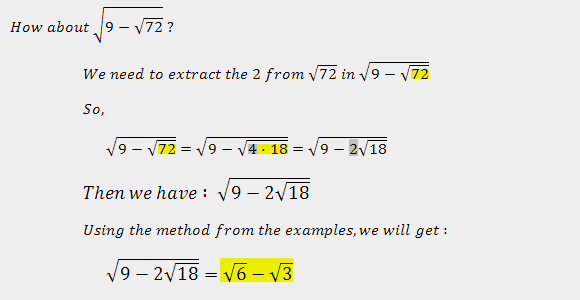## Tuesday, May 13, 2014

### Math: (Shortcut for) Simplifying (Denesting) Nested Square Roots

This is a senior high Algebra material.
If you're teaching (or tutoring) or probably still in high school, this method is kinda easy to understand. Kinda.

The basic form looks like this :Let's go to the methods and examples

The plus thingy√4 + √3 = 2 + √3

The minus thingyI forgot to put how to come up with 12 = 4 · 3 and 56 = 8 · 7.
The simplest way is to factor the number within the last square root. Then find the ones which if added will produce the first number.

For instance √(8 + 2√15)

We have 8 as the first number and 15 as the last one.
Factors of 15 :
• 1 · 15 = 15
• 3 · 5 = 15
Therefore, we choose the 2nd one, the 3 · 5 = 15
because 3 + 5 = 8
So we have x = 3 and y = 5
We put all together : √(8 + 2√15) = √3 + √5

Trick #1Trick #2If you wanna clarify the simplified forms above, you can go to Wolfram Alpha ➡ then type the complicated-er forms from my examples.

This is fun.

1.This was very useful. Thank you

1.You're welcome.

2.What if the nested radicand doesnt have any coefficient.

1.What about that? Please be more specific by writing an example problem of that, or you could link an image/url.

3.Thank you soo much, I have been looking for this solution for days!!!

Tell me what you think...